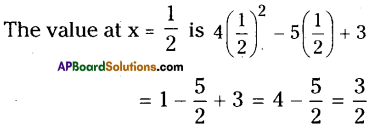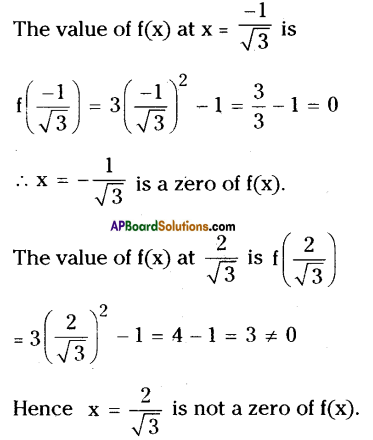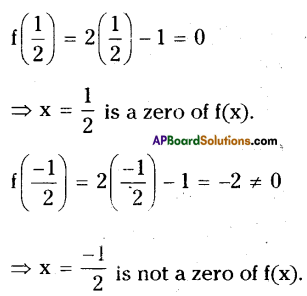AP State Syllabus AP Board 9th Class Maths Solutions Chapter 2 Polynomials and Factorisation Ex 2.2 Textbook Questions and Answers.

## AP State Syllabus 9th Class Maths Solutions 2nd Lesson Polynomials and Factorisation Exercise 2.2

Question 1.
Find the value of the polynomial 4x2 – 5x + 3, when
(i) x = 0
Solution:
The value at x = 0 is
4(0)2 – 5(0) + 3
= 3(ii) x = – 1
Solution:
The value at x = – 1 is
4 (- 1)2 – 5 (- 1) + 3
= 4 + 5 + 3
= 12

iii) x = 2
Solution:
The value at x = 2 is
4(2)2 – 5(2) + 3
= 16 -10 + 3
= 9

iv) x = $$\frac{1}{2}$$
Solution:Question 2.
Find p(0), p(1) and p(2) for each of the following polynomials.
i) p(x) = x2 – x + 1
Solution:
p(0) = 02 – 0 + 1 = 1
p(1) = 12 – 1 + 1 = 1
p(2) = 22 – 2 + 1 = 3

ii) P(y) = 2 + y + 2y2 – y3
Solution:
p(0) = 2 + 0 + 2(0)2 – 03 = 2
p(1) = 2+ 1 + 2(1)2 – 13 = 4
p(2) = 2 + 2 + 2(2)2 -23 = 4 + 8- 8 = 4iii) P(z) = z3
Solution:
p(0) = 03 = 0
p(1) = 13 = 1
p(2) = 23 = 8

iv) p(t) = (t – 1)(t + 1) = t2 – 1
Solution:
p(0) = (0 – 1) (0 + 1) = – 1
p(1) = t2 – 1 = 12 – 1 = 0
p(2) = 22 – 1 = 4 – 1 = 3

v) p(x) = x2 – 3x + 2
Solution:
p(0) = 02 – 3(0) + 2 = 2
p(1) = 12 – 3(1) + 2 = 1 – 3 + 2 = 0
p(2) = 22 – 3(2) + 2 = 4- 6 + 2 = 0

Question 3.
Verify whether the values of x given in each case are the zeroes of the polynomial or not ?
i) p(x) = 2x + 1; x = $$\frac{-1}{2}$$
Solution:
The value of p(x) at x = $$\frac{-1}{2}$$ is
$$\mathrm{p}\left(\frac{-1}{2}\right)=2\left(\frac{-1}{2}\right)+1$$
= -1 + 1 = 0
∴ x = $$\frac{-1}{2}$$ is a zero of p(x).(ii) p(x) = 5x – π ; x = $$\frac{-3}{2}$$
Solution:
The value of p(x) at x = $$\frac{-3}{2}$$ is
$$\mathrm{p}\left(\frac{-3}{2}\right)=5\left(\frac{-3}{2}\right)-\pi=\frac{-15}{2}-\pi \neq 0$$
∴ x = $$\frac{-3}{2}$$ is not a zero of p(x).

iii) p(x) = x2 – 1; x = ±1
Solution:
The value of p(x) at x = 1 and – 1 is
p(1) = 12 – 1 = 0
p(-1) = (-1)2 -1 = 0
∴ x = ±1 is a zero of p(x).

iv) p(x) = (x – 1) (x + 2); x = – 1, – 2
Solution:
The value of p(x) at x = – 1 is
p(-1) = (-1 – 1) (-1 + 2)
=-2 x 1 =-2 ≠ 0
Hence x = – 1 is not a zero of p(x).
And the value of p(x) at x = – 2 is
p (- 2) = (- 2 – 1) (- 2 + 2) = – 3 x 0 = 0
Hence, x = – 2 is a zero of p(x).

v) p(y) = y2; y = o
Solution:
The value of p(y) at y = 0 is p(0) = 02 = 0
Hence y = 0 is a zero of p(y).vi) p(x) = ax + b ; x = $$\frac{-\mathbf{b}}{\mathbf{a}}$$
Solution:
The value of p(x) at x = $$\frac{-\mathbf{b}}{\mathbf{a}}$$ is
$$\mathrm{p}\left(\frac{-\mathrm{b}}{\mathrm{a}}\right)=\mathrm{a}\left(\frac{-\mathrm{b}}{\mathrm{a}}\right)+\mathrm{b}$$
= -b + b = 0
∴ x = $$\frac{-\mathbf{b}}{\mathbf{a}}$$ is a zero of p(x).

vii) f(x) = 3x2 – 1; x = $$\frac{-1}{\sqrt{3}}, \frac{2}{\sqrt{3}}$$
Solution:viii) f(x) = 2x – 1; x = $$\frac{1}{2} ;-\frac{1}{2}$$
Solution:Question 4.
Find the zero of the polynomial in each of the following cases.
i) f(x) = x + 2
Solution:
x + 2 = 0
x = – 2

ii) f(x) = x – 2
Solution:
x – 2 = 0
x = 2

iii) f(x) = 2x + 3
Solution:
2x + 3 = 0
2x = – 3
x = $$\frac{-3}{2}$$iv) f(x) = 2x – 3
Solution:
2x – 3 = 0
2x = 3
x = $$\frac{3}{2}$$

v) f(x) = x2
Solution:
x2 = 0
x = 0

vi) f(x) = px, p ≠ 0
Solutin:
px = 0
x = 0

vii) f(x) = px + q; p ≠ 0; p, q are real numbers.
Solution:
px + q = 0
px = -q
x = $$\frac{-\mathrm{q}}{\mathrm{p}}$$Question 5.
If 2 is a zero of the polynomial p(x) = 2x2 – 3x + 7a, find the value of
a.
Solution:
Given that 2 is a zero of p(x) = 2x2 – 3x + 7a
(i.e.) p(2) = 0
⇒ 2(2)2 – 3(2) + 7a = 0
⇒ 8 – 6 + 7a = 0
⇒ 2 + 7a = 0
⇒ 7a = – 2
⇒ a = $$\frac{-2}{7}$$Question 6.
If 0 and 1 are the zeroes of the polynomial f(x) = 2x3 – 3x2 + ax + b, find the values of a and b.
Solution:
Given that f(0) = 0; f(1) = 0 and
f(x) = 2x3 – 3x2 + ax + b
∴ f(0) = 2(0)3 – 3(0)2 + a(0) + b
⇒ 0 = b
Also f(1) = 0
⇒ 2(1)3 – 3(1)2 + a(1) + 0 = 0
⇒ 2 – 3 + a = 0 .
⇒ a = 1
Hence a = 1; b = 0